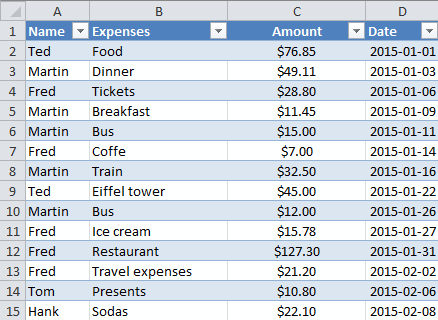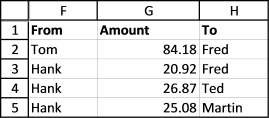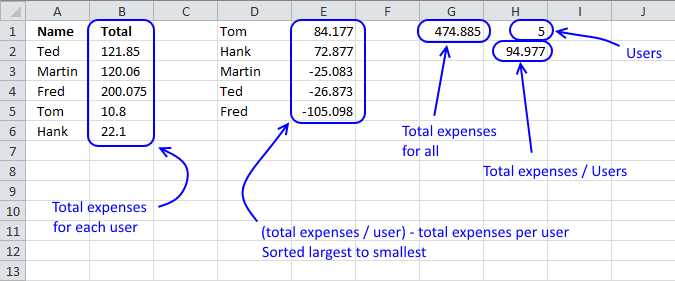Author: Oscar Cronquist Article last updated on July 31, 2017This workbook lets you split expenses evenly with other people. Type name, expense and amount in the excel table on sheet 'Expenses'.Excel returns amounts to be paid and individuals involved. This is not the macro you are looking for if you want to calculate the smallest number of transactions possible.### How calculation sheet worksThe vba macro uses the values in cell range D1:E5 and calculates transactions so all sums even out.

For example, Tom pays Fred \$84.177 and his sum is 84.177 - 84.177 = 0. Hank pays Fred \$20.92, Ted \$26.87, Martin \$25.08 and his sum is 72.88 - 20.92 - 26.87 - 25.08 = 0.

Those were the necessary transactions to even out all user sums to zero. The remaining sums are now all 0, Martin's sum is -25.08 + 25.08 = 0, Ted -26.87 + 26.87 = 0 and Fred -105.098 + 84.177 + 20.92 = 0.

### How I made this workbook

There are two sheets in this workbook, 'Expenses' and 'Calculation'. Here are the formulas on 'Calculation' sheet.

Unique distinct names in column A:

=IFERROR(INDEX(Table1[Name],MATCH(0,COUNTIF(\$A\$1:A1,Table1[Name]),0)),"")

Read this post: How to extract a unique distinct list from a column

Sum values for each unique name in column B:

=IF(A2<>"",SUMIF(Table1[Name],A2,Table1[Amount]),"")

Count unique names in cell H1:

=MAX(IF(A2:A38<>"",MATCH(ROW(A2:A38),ROW(A2:A38)),""))

Sum amounts in cell G1:

=SUM(B2:B34)

VBA macro

```Sub SplitExp()
Dim r As Single, d As Single, e As Single, Lrow As Single
Application.ScreenUpdating = False
With Worksheets("Calculation")
r = .Range("H1")
.Columns("D:E").Clear
Worksheets("Expenses").Range("F2:H100").Clear
.Range("D" &amp; 1 &amp; ":E" &amp; r) = .Range("A" &amp; 2 &amp; ":B" &amp; r + 1).Value
For d = 1 To r
.Range("E" &amp; d) = (.Range("G1") / r) - .Range("E" &amp; d)
Next d
For d = 1 To r
For e = r To 1 Step -1
Lrow = Worksheets("Expenses").Range("F" &amp; Rows.Count).End(xlUp).Row + 1
If Round(.Range("E" &amp; d), 2) &lt;&gt; 0 And Round(.Range("E" &amp; e), 2) &lt;&gt; 0 Then
If Application.Min(Abs(.Range("E" &amp; d)), Abs(.Range("E" &amp; e))) = Abs(.Range("E" &amp; d)) Then
Worksheets("Expenses").Range("F" &amp; Lrow &amp; ":H" &amp; Lrow) = Array(.Range("D" &amp; d), Round(Abs(.Range("E" &amp; d)), 2), .Range("D" &amp; e))
.Range("E" &amp; e) = .Range("E" &amp; e) + .Range("E" &amp; d)
.Range("E" &amp; d) = 0
Else
Worksheets("Expenses").Range("F" &amp; Lrow &amp; ":H" &amp; Lrow) = Array(.Range("D" &amp; d), Round(Abs(.Range("E" &amp; e)), 2), .Range("D" &amp; e))
.Range("E" &amp; d) = .Range("E" &amp; e) + .Range("E" &amp; d)
.Range("E" &amp; e) = 0
End If
End If
Next e
Next d
End With
Application.ScreenUpdating = True
End Sub

```

Event code, sheet 'Calculation'

```Private Sub Worksheet_Calculate()

Call SplitExp

End Sub
```